# Leakage Inductance Part II

The non-linked flux between primary and secondary windings of transformers leads to what is commonly known as leakage inductance. Its magnitude plays a key role in modern switched-mode power supplies. Whether it needs to be minimized or maximized, the use of complex prediction models, that are many times far from reality, is required.

The objective of this paper is to provide a general idea of the leakage inductance range values found in different topologies and turns ratios.

## Case of Study

As explained in previous application notes, two of the key parameters affecting the leakage inductance are the transformer turns ratio and winding arrangement. With the aim of having a reference when creating a design, a brief summary of the leakage inductance measured will be provided for different transformer designs measured at Frenetic’s Laboratory.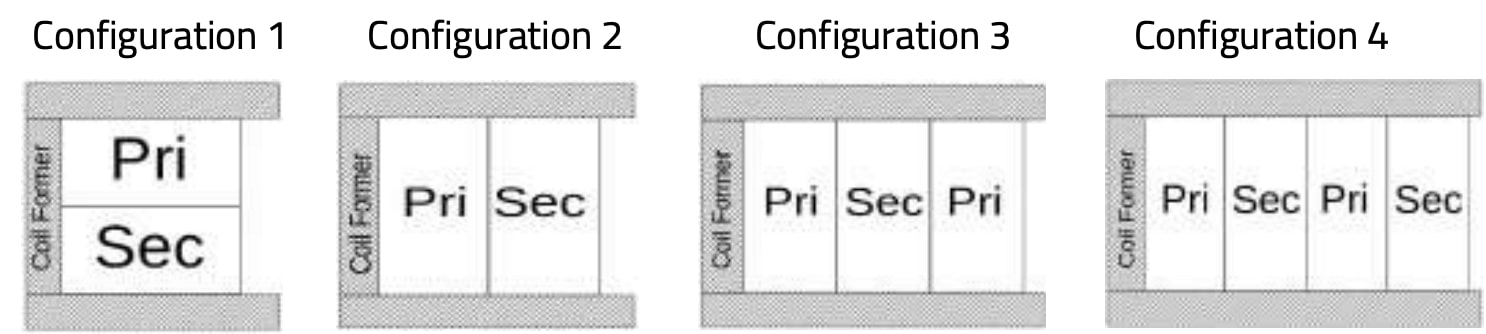Figure 1: Winding arrangement configurations used for the measurements.

Values of leakage inductance will be measured for different converters with four different winding configurations, from the high-pot winding arrangement (Conf. 1) to two layers interleaving arrangement (Conf. 4) passing through simple P-S configuration (Conf. 2) and simple interleaving (Conf. 3).

## Experimental Results:

Table 1 shows the converters used for this experiment for each topology with different configurations of windings. Results demonstrate how the smaller the difference between primary and secondary turns, and the better the interleaving arrangement, the smaller the leakage inductance achieved.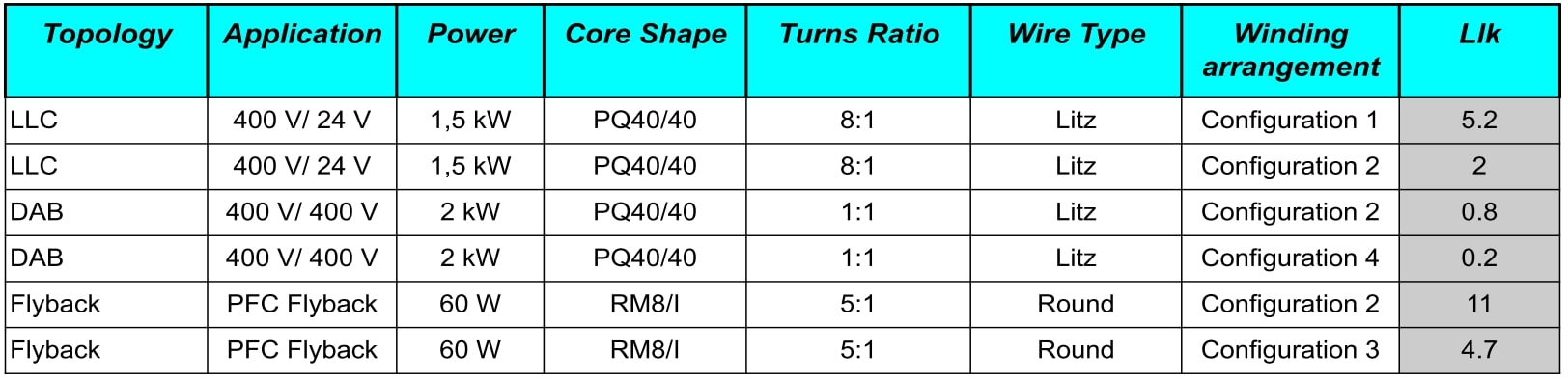Table 1: Data of the different converters used in the experiment

Case 1

Figure 2 shows how the leakage inductance is reduced by 50% from Conf. 1 to Conf. 3 in an RM8/I. The more is the winding interleaved, the lower the leakage. Minimization of the leakage inductance in flyback converters is generally a must. Otherwise high voltage spikes at the switching node would be present with the increased stress that this involves in the semiconductors. The interleaved planar transformer could be used in this case but taking special care of the parasitic capacitance that will create a path for common-mode noise.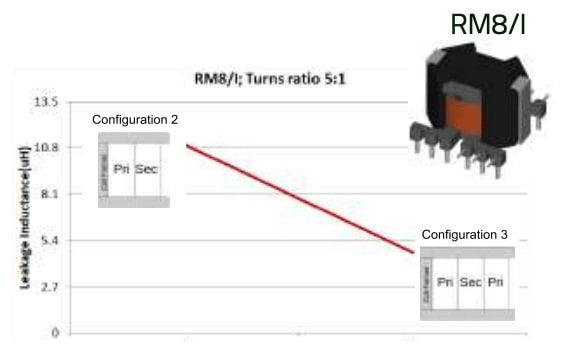Figure 2: Leakage inductance in two RM8/I with a turns ratio of 5:1.

Case 2

In Figure 3, we used a PQ40/40 with a turn ratio of 1:1. The Llk is reduced by a factor of 4. The decrease in the leakage inductance appears as a consequence of the division of the winding on a higher amount of layers with interleaving arrangement, from P-S to P-S-P-S arrangement.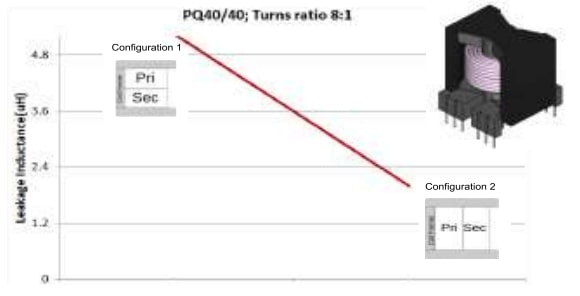Figure 3: Leakage inductance in two PQ40/40 with a turns ratio of 1:1.

Case 3

Case 3 uses the same core shape as in case 2 but with 8 times higher turns ratio (8:1). Winding Conf. 1 and 2 do not seem very different, however, as we can see in the results, the Llk is reduced 2.6 times, from 5.2 to 2 uH. Comparing to case 2, it seems to have lower Llk change, but talking in absolute values it has a higher impact, as in Case 2 the leakage is reduced 0.6 uH, and in Case 3 is of 3.2 uH.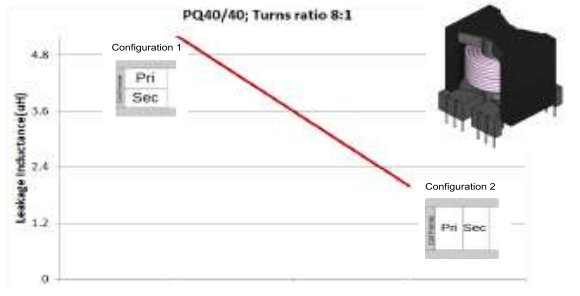Figure 4: Leakage inductance in two PQ40/40 with a turns ratio of 8:1.

### Conclusions

From the results shown on the experimental cases, it can be concluded that as the turns ratio gets closer to 1, the coupling between windings leads to leakage inductances below 1 uH. In cases where the turns ratio is higher, the winding configuration is of much importance to reduce the leakage inductance, however, it will be still in considerable ranges of inductance, it can be reduced only up to a certain point.

Do you want the full version?